# BJT Q-Point

#### SamR

Joined Mar 19, 2019
4,613
Here is what I am given. β= 200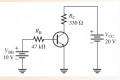From that I calculated:
$$I_B = (V_{BB} - V_{BE})/R_B = (10V - 0.7V)/47kΩ = 197.87uA$$
$$I_C = ßI_B = 200*197.87uA = 39.57mA$$
$$V_{CE} = V_{CC} - I_CR_C = 6.94V$$
$$I_{Csat} = (V_{CC} - V_{CEsat})/R_C = 60.6mA$$
$$(I\, assumed \, 0\, for\, V_{CEsat})$$

Up to this point I am OK. Then they gave me this: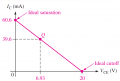They are using the calculated $$I_C$$ for the Q-Point. Looking at the Load line graph, I would think the Q-Point would be where the 10V intersects the mA on the DC Load Line? Why would the Q-Point not be in the center of the DC Load Line?

#### Papabravo

Joined Feb 24, 2006
20,142
I think the Q-point is at the collector current determined by Vcc and Rc with Vce(sat) = 0. The existence of the load line implies that Ic and Vce are not independent. Once you determine Ic, the corresponding Vce must be on the load line. It should be evident that you can determine the equation of the load line which has a fixed slope (-1/Rc) and y-intercept (Ic=60.6 mA). Therefore, Ve is determined by the equation of the load line.

$$I_{C}\;=\;60.6\text{ ma}\;-\;\left(\cfrac{V_{CE}}{R_{C}}\right)$$
$$I_{C}\;=\; 60.6\text{ ma}\;-\;\left(\cfrac{6.93\text{ V}}{330\text{ Ω}}\right)\;\approx\;39.6\text{ ma}$$

You can also rewrite the equation of the load line by solving for Vce. It tlooks like this:

$$V_{CE}\;=\;330\text{ Ω}\times(60.6\text{ ma}\;-\;39.6\text{ ma})\;=\;6.93\text{ V}$$

This is the same logic that tells you why you cannot build a power supply where current into and voltage across the load are controlled independently.

Last edited:
•SamR

#### WBahn

Joined Mar 31, 2012
28,485
What's magical about 10 V? Just because it is half of Vcc?

Remember what the Q-point is. It is the DC operating point of the circuit with no signal applied. You calculated that directly by using the nominal current gain of the transistor.

If you are wanting to use intersecting lines on the load-line to find the Q-point, then what you need to plot are the voltage-current lines for the same two points on the circuit for two different parts of the circuit. The straight line on the graph is the V-I charactersitic for Ic vs Vce as imposed by the Vcc and Rc components. Next you would plot the Icc vs Vce as imposed by the transistor. The Q-point would be where those two plots interset.

But you don't need to do that because you calculated it directly.

•Papabravo

#### SamR

Joined Mar 19, 2019
4,613
I'm thinking ahead to the ac signal. Placing the Q-point at center allows a greater ac signal amplitude without saturation or cutoff clipping? I'm simply using what I was given. At this point, the AC Load Line has not been introduced yet. It just seems counterintuitive that the midpoint of the load line would not be used as the Q-Point. Then again, why use 20V Vcc?

using the nominal current gain of the transistor
Without using an actual component and its rated specifications, the 200 β is simply an illustration. If I read your answers correctly, the Ic value is the key and not the voltage? Which would mean the 20Vcc is a bit unnecessarily excessive? I still want to see the Q-Point positioned to avoid ac signal clipping, or think I do.

Last edited:

#### Papabravo

Joined Feb 24, 2006
20,142
I'm thinking ahead to the ac signal. Placing the Q-point at center allows a greater ac signal amplitude without saturation or cutoff clipping? I'm simply using what I was given. At this point, the AC Load Line has not been introduced yet. It just seems counterintuitive that the midpoint of the load line would not be used as the Q-Point. Then again, why use 20V Vcc?
Depends entirely on the application where the Q-point is located. Various types of amplification can happen regardless of where the Q-point is located. This is true of both Class B and Class C amplifiers. There may be reasons to avoid having a maximum swing because the next things to happen are clipping and distortion.

•SamR

#### WBahn

Joined Mar 31, 2012
28,485
I'm thinking ahead to the ac signal. Placing the Q-point at center allows a greater ac signal amplitude without saturation or cutoff clipping? I'm simply using what I was given. At this point, the AC Load Line has not been introduced yet. It just seems counterintuitive that the midpoint of the load line would not be used as the Q-Point. Then again, why use 20V Vcc?

Without using an actual component and its rated specifications, the 200 β is simply an illustration. If I read your answers correctly, the Ic value is the key and not the voltage? Which would mean the 20Vcc is a bit unnecessarily excessive? I still want to see the Q-Point positioned to avoid ac signal clipping, or think I do.
You may WANT to place the Q-point at the center, but the question doesn't ask where you want the Q-point to be, it asks where the Q-point is.

If you want to position the Q-point at the center, then you need to design the circuit so that it does so. There are several ways to do that for this circuit topology. The two obvious ones are to change the value of one (or both) resistors to place the Q-point at half the Vcc supply voltage. You could also change the value of Vcc to achieve this goal, but that is more unrealistic.

Also, note that in this circuit the Q point is strongly dependent on the beta of the transistor. This screams out that this is a very, very bad circuit design. It's fine for teaching basic analysis and even rudimentary design techniques, but any practical circuit that is strongly dependent on beta should be rejected out of hand with very, very few exceptions.

•Papabravo and SamR

#### LvW

Joined Jun 13, 2013
1,645
Also, note that in this circuit the Q point is strongly dependent on the beta of the transistor. This screams out that this is a very, very bad circuit design. It's fine for teaching basic analysis and even rudimentary design techniques, but any practical circuit that is strongly dependent on beta should be rejected out of hand with very, very few exceptions.
Yes, full agreement. The shown circuit is a very bad design.

#### Danko

Joined Nov 22, 2017
1,608
I'm thinking ahead to the ac signal. Placing the Q-point at center allows a greater ac signal amplitude without saturation or cutoff clipping?
Sometimes it is true.
You can analyze regime of transistor Q1 in circuit below.
VCC for Q1 is 11.3 V (12V - BE voltage of Q2).
Q-point is 5.31V, Ic=0.655mA.
Q1 current gain is 109, voltage gain is 4000.
Transistors Q2 and Q3 are current amplifier
with voltage gain <1.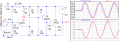#### Attachments

• 2.7 KB Views: 4
Last edited:
•SamR

#### SamR

Joined Mar 19, 2019
4,613
I prefer Floyd over some other authors for his more hands-on approach. That said, some of the teaching exercises leave me asking questions as they don't seem very practicable ... Am i correct in thinking here that the Ic value is the key and not the voltage? Or have I missed the point entirely?

Also @Danko what is the purpose of the switching diode in that circuit?

It seems I jumped the gun a bit. Later on in the exercise they use the "offset" Q-Point to illustrate "Therefore, the limiting excursion is 21 mA because the Q-point is closer to saturation than to cutoff. The 21 mA is the maximum peak variation of the collector current. Actually, it would be slightly less in practice because VCE(sat) is not quite zero." Which validates my thinking that the Q-Point should be close to the center point of the load line.

Last edited:

#### Harry Trietley

Joined Jun 29, 2022
10
Just a side note - transistor beta is a nominal figure, not at all precise. For example, 2N3904 which I've used, literally, for decades - the spec sheets lists beta (DC Current Gain) at 10 mA collector current, as being 100 min, 300 max. At 1mA it is 70 min (no max specified). At 100 mA it is 30 min (no max). These figures are from the OnSemi data sheet.
You need different circuitry to accurately set the operating point regardless of beta variations. The design would depend on what you're trying to do.

•SamR and Papabravo

#### Papabravo

Joined Feb 24, 2006
20,142
I prefer Floyd over some other authors for his more hands-on approach. That said, some of the teaching exercises leave me asking questions as they don't seem very practicable ... Am i correct in thinking here that the Ic value is the key and not the voltage? Or have I missed the point entirely?

Also @Danko what is the purpose of the switching diode in that circuit?

It seems I jumped the gun a bit. Later on in the exercise they use the "offset" Q-Point to illustrate "Therefore, the limiting excursion is 21 mA because the Q-point is closer to saturation than to cutoff. The 21 mA is the maximum peak variation of the collector current. Actually, it would be slightly less in practice because VCE(sat) is not quite zero." Which validates my thinking that the Q-Point should be close to the center point of the load line.
Basic definition of amplifier classes
• Class A Power amplifier − When the collector current flows at all times during the full cycle of signal, the power amplifier is known as class A power amplifier.
• Class B Power amplifier − When the collector current flows only during the positive half cycle of the input signal, the power amplifier is known as class B power amplifier.
• Class C Power amplifier − When the collector current flows for less than half cycle of the input signal, the power amplifier is known as class C power amplifier.

This is probably the case for a Class A amplifier which implies conduction over 360° of the input cycle. In the case of Classes B and C, the Q point is likely to be close to or inside the cutoff region, far away from the midpoint of the load line.

•SamR

#### SamR

Joined Mar 19, 2019
4,613
Still working my way up to amplifiers. Have done the basics but this book goes a bit more in depth. I'll get there...

#### Danko

Joined Nov 22, 2017
1,608
Am i correct in thinking here that the Ic value is the key and not the voltage?
Vc and Ic are interconnected.
On simulated example from post #1 you can see that range of input signal between cut off (0.7 V) and saturation (17.7 V) is 17 V.
Q-point is Vc value, which correspond to 17 V/2 + 0.7 V = 9.2 V of input signal.
So, Q-point voltage is 8.47 V: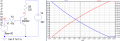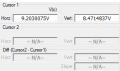•